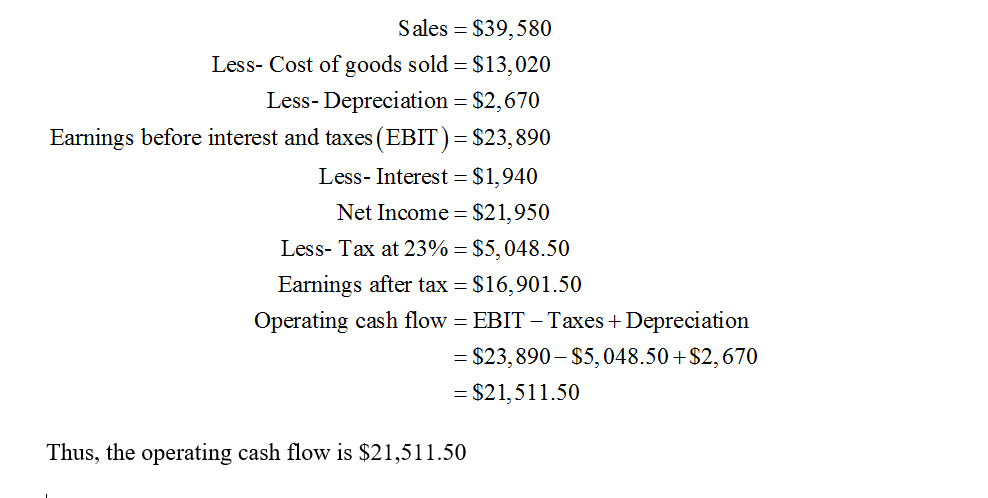10
1
watching
2,475
views

# Benson Inc has sales of \$39580 costs of \$13020, depreciation expense of \$2670, and interest expense of \$1940. The tax rate is 23 percent. What is the operating cash flow?

To calculate the operating cash flow, we need to start with the earnings before interest and taxes (EBIT) and then adjust for non-cash expenses and changes in working capital.

EBIT = Sales - Costs - Depreciation expense - Interest expense

EBIT = \$39,580 - \$13,020 - \$2,670 - \$1,940

EBIT = \$22,950

Next, we need to adjust for non-cash expenses. In this case, the only non-cash expense is depreciation:

Operating income before changes in working capital = EBIT + Depreciation

Operating income before changes in working capital = \$22,950 + \$2,670

Operating income before changes in working capital = \$25,620

Finally, we need to adjust for changes in working capital. Since the problem does not provide any information on changes in working capital, we assume that there are no changes.

Therefore, the operating cash flow is equal to the operating income before changes in working capital:

Operating cash flow = \$25,620

Thus, the operating cash flow of Benson Inc is \$25,620.

The Operating Cash Flow for Benson Inc is \$31770

Step-by-step explanation:

The operating cash flow can be calculated by subtracting Operating Expenses (costs, depreciation, and interest) from Operating Income (sales).

Operating Income = Sales - Operating Expenses Operating Expenses = Costs + Depreciation + Interest

Substituting the given values:

Operating Income = \$39580 - (\$13020 + \$2670 + \$1940) = \$31770

Therefore, the Operating Cash Flow for Benson Inc is \$31770.

Step-by-step explanation:
Operating Cash Flow = Net Income + Non-cash Expenses

Net Income = Sales - Costs - Depreciation Expense - Interest Expense - Tax Expense

Tax Expense = Tax Rate x (Sales - Costs - Depreciation Expense - Interest Expense)

Operating Cash Flow = (Sales - Costs - Depreciation Expense - Interest Expense) - (Tax Rate x (Sales - Costs - Depreciation Expense - Interest Expense)) + Depreciation Expense + Interest Expense

Operating Cash Flow = \$39580 - \$13020 - \$2670 - \$1940 - (0.23 x (\$39580 - \$13020 - \$2670 - \$1940)) + \$2670 + \$1940

Operating Cash Flow = \$22,450

Step-by-step explanation:

Operational cash flow

Sales.               =\$39580

-cost.               =\$13020

-depreciation. =\$2670

Operating income=\$23890

-interest expense=\$1940

income before tax=\$21950

-tax=23%=0.23*21950=\$5048.5

Tax income=\$16901.5

Operational cash flow=Net income+non-cash expenses-increase in working capital

Operating cash Flow=\$16901.5+\$2670-0

Operating Cash flow=\$19571.5

\$19,571.50

Step-by-step explanation:
Operating cash flow generated by the company from its operations, in other words, the number of cash resources derived from operating activities, which is the total cash flow that would be shown under the operating activities section of the cash flow statement.

operating cash flow=net income+depreciation

The depreciation would be added back to the net income in a bid to determine the operating cash flow since it is a non-cash charge

Earnings before tax=sales-costs-depreciation-interest expense

Earnings before tax=\$39580-\$13,020-\$2670-\$1940

Earnings before tax=\$21,950

Net income=earnings before tax*(1-tax rate)

tax rate =23%

net income=\$21950*(1-23%)

net income=\$16,901.50

depreciation=\$2670

operating cash flow=\$16,901.50+\$2670

operating cash flow=

\$19,571.50Operating Cash flow is \$19,440

Explanation:

Operating Cash flow

Sales                         = \$38,530

-Costs                        = \$12,750

-Depreciation            = \$2,550

Operating Income    = \$23,230

-Interest Expense     = \$1,850

Income before Tax  = \$21,380

-Tax                           = \$4,490

Net Income               = \$16,890

Operating Cash Flow = Net Income + Non-Cash Expenses – Increase in Working Capital

Operating Cash flow = \$16,890 + \$2,550 - 0

Operating Cash flow = \$19,440

21,511.5

Step-by-step explanation:

Sales = \$ 39,580

Less: Cost = \$ 13,020

EBIDT = \$ 26,560

less: Depreciation = \$ 2,670

EBIT = \$ 23,890

less: Interest = \$ 1,940

EBT = \$ 21,950

less: Taxes = \$ 5,048.5 ( \$ 21,950 * 23%)

Net Income = \$ 16,901.50

Operating cash flow (OCF) = EBIT + depreciation - taxes

= \$ 23,890 + \$ 2,670 - \$ 5,048.5

= \$ 21,511.5Step-by-step explanation:
Based on indirect method of calculation, operating cash flow is net income adjusted for non-cash items like depreciation, AR and AP.

Profit before tax = Sales - costs - depreciation - interest = 39,580 - 13,020 - 2,670 - 1,940 = 21,950

Net Income = Profit before tax - tax = 21,950 - 23%*21,950 = 16,901.5

Operating cash flow = Net income + depreciation = 16,901.5 + 2,670 = 19,571.5Operating Cash flow=\$19571.5
Step-by-step explanation:
Operational cash flow

Sales.               =\$39580

-cost.               =\$13020

-depreciation. =\$2670

Operating income=\$23890

-interest expense=\$1940

income before tax=\$21950

-tax=23%=0.23*21950=\$5048.5

Tax income=\$16901.5

Operational cash flow=Net income+non-cash expenses-increase in working capital

Operating cash Flow=\$16901.5+\$2670-0

Operating Cash flow=\$19571.5